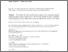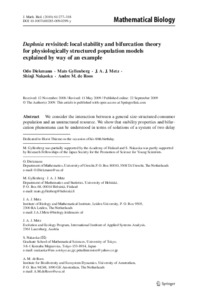# Daphnia revisited: Local stability and bifurcation theory for physiologically structured population models explained by way of an example

Diekmann, O., Gyllenberg, M., Metz, J.A.J., Nakaoka, S., & de Roos, A.M. (2010). Daphnia revisited: Local stability and bifurcation theory for physiologically structured population models explained by way of an example. Journal of Mathematical Biology 61 (2) 277-318. 10.1007/s00285-009-0299-y.Preview
Text
s00285-009-0299-y.pdf - Published Version

## Abstract

We consider the interaction between a general size-structured consumer population and an unstructured resource. We show that stability properties and bifurcation phenomena can be understood in terms of solutions of a system of two delay equations (a renewal equation for the consumer population birth rate coupled to a delay differetial equation for the resource concentration). As many results for such systems are available, we can draw rigorous conclusions concerning dynamical behaviour from an analysis of a characteristic equation. We derive the characteristic equation for a fairly general class of population models, including those based on the Kooijman-Metz Daphnia model and a model introduced by Gurney-Nisbet and Jones et al., and next obtain various ecological insights by analytical or numerical studies of special cases.

Item Type: Article Physiologically structured population models; Size-structure; Delay equations; Linearised sability; Characteristic equation Evolution and Ecology (EEP) Publication - http://pure.iiasa.ac.at/14731/ IIASA Import 15 Jan 2016 08:44 27 Aug 2021 17:21 http://pure.iiasa.ac.at/9250View Item